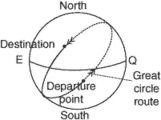geodesic

(redirected from Geodesic equation)
Also found in: Dictionary, Thesaurus.

geodesic

1. relating to or involving the geometry of curved surfaces
2. the shortest line between two points on a curved or plane surface
Collins Discovery Encyclopedia, 1st edition © HarperCollins Publishers 2005

geodesic

[¦jē·ə¦des·ik]
(mathematics)
A curve joining two points in a Riemannian manifold which has minimum length.
McGraw-Hill Dictionary of Scientific & Technical Terms, 6E, Copyright © 2003 by The McGraw-Hill Companies, Inc.

geodesicGreat circle is the shortest distance between two points.
The shortest distance between two points on any surface. The shortest distance between two places on earth derived mathematically is called a geodetic line.
References in periodicals archive ?
Let us consider two neighboring geodesic paths [[theta].sup.[alpha]]([tau]) and [[theta].sup.[alpha]]([tau]) + [delta][[theta].sup.[alpha]]([tau]), with [tau] denoting the affine parameter, that satisfy the following geodesic equations of motion:
For the geodesic equation in this notation we have [F.sup.i] = - [[GAMMA].sup.i.sub.[mu]v]([v.sup.[mu]][v.sup.v]/([c.sup.2]-v[gamma]v)).
In two recent papers [4, 5], I solved the scalar geodesic equation for mass-bearing particles and massless particles (photons), in the most studied particular spaces: in the space of Schwarzschild's mass-point metric, in the space of an electrically charged mass-point (the Reissner-Nordstrom metric), in the rotating space of Godel's metric (a homogeneous distribution of ideal liquid and physical vacuum), in the space of a sphere of incompressible liquid (Schwarzschild's metric), in the space of a sphere filled with physical vacuum (de Sitter's metric), and in the deforming space of Friedmann's metric (empty or filled with ideal liquid and physical vacuum).
The second problem was solved using the geodesic equations for light-like particles (photons) which are mediators for electromagnetic radiation.
whose Euler-Lagrange equations are the geodesic equations
* We should also add that, coming from the geodesic equation along the z-axis, which is the third equation of (61), to the simplified form (62) thereof, we omitted the harmonic term from consideration.
If a geodesic equation must be produced, for a monochromatic wave with frequency w, the form of a photonic energy tensor should be similar to that of massive matter.
From the geodesic equation, clearly it is impossible to justify (7) for any frame of reference.
(3) allows [[nabla].sup.c] T[(L).sub.cb] = 0 to generate the necessary geodesic equation for photons.
which is different from the geodesic equation in that the right part in not zero here.
In order to study the motion of planets and light rays in a homogeneous time varying spherical spacetime, there is need to derive the geodesic equations .

Site: Follow: Share:
Open / Close